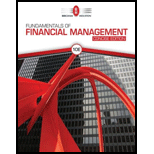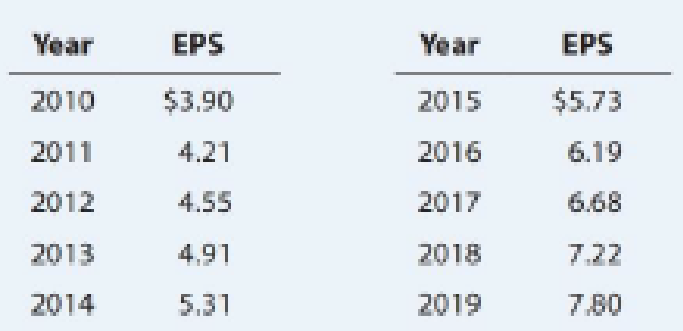# WACC The following table gives Foust Company’s earnings per share for the last 10 years. The common stock, 7.8 million shares outstanding, is now (1/1/20) selling for $65.00 per share. The expected dividend at the end of the current year (12/31/20) is 55% of the 2019 EPS. Because investors expect past trends to continue, g may be based on the historical earnings growth rate. (Note that 9 years of growth are reflected in the 10 years of data.) The current interest rate on new debt is 9%; Foust’s marginal tax rate is 25%, and its target capital structure is 40% debt and 60% equity. a. Calculate Foust’s after-tax cost of debt and common equity. Calculate the cost of equity as r s = D 1 /P 0 + g. b. Find Foust’s WACC.BuyFindarrow_forward ### Fundamentals Of Financial Manageme... 10th Edition Eugene F. Brigham + 1 other Publisher: Cengage Learning ISBN: 9781337902571 #### Solutions Chapter SectionBuyFindarrow_forward ### Fundamentals Of Financial Manageme... 10th Edition Eugene F. Brigham + 1 other Publisher: Cengage Learning ISBN: 9781337902571 Chapter 10, Problem 20P Textbook Problem 170 views ## WACC The following table gives Foust Company’s earnings per share for the last 10 years. The common stock, 7.8 million shares outstanding, is now (1/1/20) selling for$65.00 per share. The expected dividend at the end of the current year (12/31/20) is 55% of the 2019 EPS. Because investors expect past trends to continue, g may be based on the historical earnings growth rate. (Note that 9 years of growth are reflected in the 10 years of data.)The current interest rate on new debt is 9%; Foust’s marginal tax rate is 25%, and its target capital structure is 40% debt and 60% equity. a. Calculate Foust’s after-tax cost of debt and common equity. Calculate the cost of equity as rs = D1/P0 + g. b. Find Foust’s WACC.

a.

Summary Introduction

To identify: The after-tax cost of debt and cost of common equity.

After-Tax Cost of Debt

It can be defined as a relevant cost of the new debt by considering the tax deductibility in interest. It is the cost of the debt after tax savings.  The interest on the debt is tax deductible. The tax can saved on the interest paid on the debt. It is used to calculate the weighted average cost of capital.

Cost of Equity

It is the cost of the company while raising finance by issuing equity. It is the earnings from the investment to the firm’s equity investors. It is the return to the stockholders’ equity investments. The issue of new stock incurs the flotation cost.

Cost of Capital:

In any company there is a need of the fund for various purposes. The companies raise capital through various sources such as equity, debt, and preferred stock. The cost of capital is the total cost of raising capital. It consists of the cost of debt, cost of equity, and cost of preferred stock.

### Explanation of Solution

The formula to calculate cost of the equity is as follows:

r=D1P0+g

Where,

• D1 is the next expected dividend.
• P0 is the current market price.
• g is the growth rate.

Substitute $4.29 for D1,$65 for P0, 0.08 (working notes) for g in above formula.

r=$4.29$65+0.08=$4.29$65+0.08=0.066+0.08=14.6%

Thus, the cost of equity is 14.6%.

Working note:

Compute growth rate.

Given,

EPS in year 2010 is $3.90. EPS in year 2019 is$7

b.

Summary Introduction

To determine: WACC of F Company.

Weighted Average Cost of Capital

It is the weighted average cost of capital of all the sources through which the firm finances its capital. It is the rate that a company will pay to all for raising finance. It can be termed as the firm’s cost of capital. The company raises money through various sources such as common stock and preference share debt. The WACC is calculated by taking the relative weight of each item of capital structure.

### Still sussing out bartleby?

Check out a sample textbook solution.

See a sample solution

#### The Solution to Your Study Problems

Bartleby provides explanations to thousands of textbook problems written by our experts, many with advanced degrees!

Get Started

Find more solutions based on key concepts
What role do past costs play in relevant costing decisions?

Managerial Accounting: The Cornerstone of Business Decision-Making

EXPECTATIONS THEORY One-year Treasury securities yield 5%. The market anticipates that 1 year from now, 1-year ...

Fundamentals of Financial Management, Concise Edition (with Thomson ONE - Business School Edition, 1 term (6 months) Printed Access Card) (MindTap Course List)

DETERMINING DUE DATE Determine the due date for the following notes. (Assume there are 28 days in February.)

College Accounting, Chapters 1-27 (New in Accounting from Heintz and Parry)